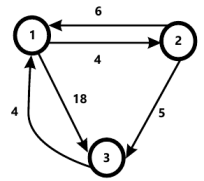MORE IN Analysis of Algorithms
MU Computer Engineering (Semester 4)
Analysis of Algorithms
December 2012
Total marks: --
Total time: --
INSTRUCTIONS
(1) Assume appropriate data and state your reasons
(2) Marks are given to the right of every question
(3) Draw neat diagrams wherever necessary

1 (a) Arrange the following functions in increasing order
N, log n, n3, n2, nlogn, 2n, n!
5 M
1 (b) Explain general method of dynamic programming. List different examples of it.
5 M
1 (c) Justify Greedy approach for solving knapsack problem.
5 M
1 (d) State Graph Colouring problem. State the strategy used to solve above problem.
5 M

2 (a) Sort the following numbers using Merge Sort-
27, 6, 18, 25, 48, 59, 98, 34.
Give the output of each pass. Write an algorithm for merge sort.
10 M
2 (b) Write an algorithm for minimum spanning tree using Prim's method.
10 M

3 (a) Explain N-Queen's problem using backtracking with algorithm.
10 M
3 (b) Consider the following set of frequencies
A=2, B=6, C=4, D=15, E=7, F=22, H=17
Find Huffman codes for the same.
10 M

4 (a) Describe 0/1 knapsack problem. How to solve it using branch and bound?
10 M
4 (b) Write an algorithm for binary search. Derive its best case and worst case complexities.
10 M

5 (a) For the following graph find all pairs shortest path using dynamic programming.10 M
5 (b) Explain optimal storage on tapes.
10 M

6 (a) Find longest subsequence of given two steps
X = {B A T A}
Y = {T A T A}
10 M
6 (b) Explain single source shortest path using dynamic programming. Write an algorithm for the same.
10 M

Write short notes on the following :-